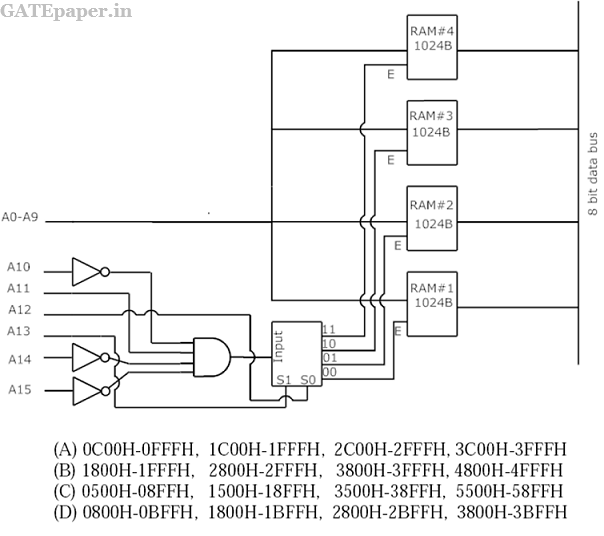### GATE 2013 ECE Video Solution on Digital Circuits (Digital Electronics)

1. A bulb in a staircase has two switches, one switch being at the ground floor and the other one at the first floor. The bulb can be turned ON and also can be turned OFF by any one of the switches irrespective of the state of the other switch. The logic of switching of the bulb resembles
a. An AND gate
b. An OR gate
c. An XOR gate
d. A NAND gate

2. For 8085 microprocessor, the following program is executed.
MVI A,05H
MVI B, 05H
DCR B
JNZ PTR
HLT
At the end of the program, accumulator contains
a. 17H
b. 20H
c. 23H
d. 05H

3. In the circuit shown below, Q1 has negligible collector to emitter saturation voltage and the diode drops negligible voltage across it under forward bias. If VCC is +5 volts, X and Y are digital signals with 0 volts as logic 0 and VCC as logic 1, then the Boolean expression for Z is4. There are four chips each of 1024 bytes connected to a 16 bit address bus as shown in the figure below. RAMs 1,2,3 and 4 respectively are mapped to addresses